# Bitwise Operators in C++

## Bitwise Operators in C++ with Examples:

In this article, I am going to discuss Bitwise Operators in C++ with Examples. Please read our previous article where we discussed Overflow in C++ with Examples.

##### Bitwise Operators in C++:

These operations are performed on the bits of the data not on the whole data as on a single unit but on the bits of the data. Bitwise operators operate on bits and it performs a bit-by-bit operation. The Bitwise operators in C++ are as follows.

1. Bitwise And &
2. Bitwise OR |
3. Bitwise X-OR ^
4. Bitwise Not ~
5. Binary Leftshift <<
6. Binary Rightshift >>

Let us see the operations available, bitwise AND, bitwise OR, bitwise XOR, bitwise NOT, left shift, and right shift.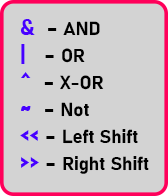Let me quickly show you how these operations work.

##### Bitwise AND:

Suppose if there are two bits, bit 1 and bit 2, Two-Bits are there then:

1. If bit 1 and bit 2 both are 0 then bit 1 & bit 2 will also be 0.
2. If bit 1 is 1 and bit 2 is 0 then bit 1 & bit 2 will be 0.
3. If bit 1 is 0 and bit 2 is 1 then bit 1 & bit 2 will be 0.
4. If bit 1 and bit 2 both are 1 then only bit 1 & bit 2 will be 1.

You can see the table below: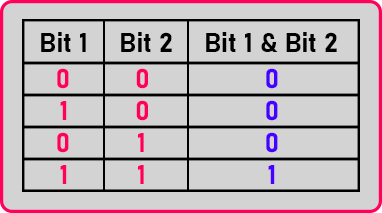It is just like a logical AND. So, when we use a bit-wise AND it will be 1 if both the bits are 1.

##### Bitwise OR:

Then if we talk about OR then: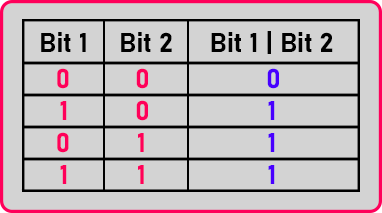Here we can see in the table that Bit 1 | Bit 2 will be 1 if any one of the bits is 1. And Bit 1 | Bit 2 will be 0 if both the bits are 0.

##### Bitwise XOR (Exclusively-OR):

Now if it is XOR then,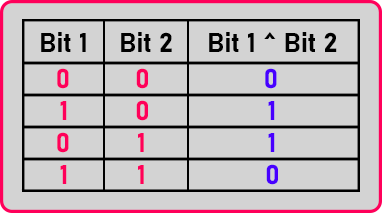Then, if both the bits are different then only Bit 1 ^ Bit 2 will be 1. If Bit 1 and Bit 2 are the same then Bit 1 ^ Bit 2 will be 0. The other bit must be 0 or if one bit is 0 then the other bit must be 1 then only Bit 1 ^ Bit 2 will be 1. Let us learn all these operations by using examples.

int x = 11, y = 5, z;

We have an integer variable ‘x’ of value 11, another variable ‘y’ of value 5, and ‘z’. We know that these numbers are actually stored in binary form. So, the value of ‘x’ and ‘y’ in the binary form will be: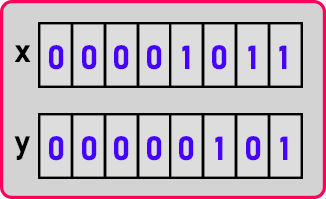We have assumed that int takes 2 bytes means 16 bits but here we have shown only 8 bits as all remaining bits are ‘0’.

##### Bitwise NOT (Compliment):

NOT is a unary operation that means an operation with only one operand. In this operation,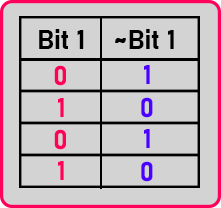Here you can see that the NOT inverts the bits of its operant. If the bit is 1 then NOT will be 0 and if the bit is 0 then NOT will be 1.

##### Bitwise AND Example:

Now If I use AND operation and store the result in variable ‘z’ then,

int x = 11, y = 5, z;

z = x & y;

So, the bits of ‘x’ and ‘y’ will be AND. Above we see the truth table and according to that we will apply AND between x and y as: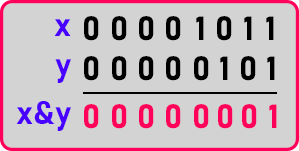The result of x&y will be ‘0 0 0 0 0 0 0 1’. It is ‘1’ in decimal form so ‘1’ will be stored in the ‘z’ variable. So, in this way, we performed Bitwise AND operation.

##### Bitwise OR Example:

Let us see what will be the result of OR operation.

int x = 11, y = 7, z;

Here we have taken ‘x’ as 11, ‘y’ as 7, and z uninitialized. Now we will use show you OR operation between x and y: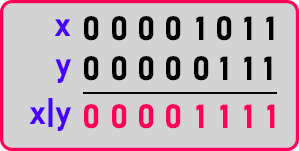Here the result will be one if either of any bit is 1. The result of x|y is ‘0 0 0 0 1 1 1 1’. It is 15 in decimal form and this 15 will be stored in the ‘z’ variable. It is not like addition or subtraction that we directly look at the value and give the answer. Unless we perform some bitwise operations, we cannot get the answer. So, if you have some practice on this then you may be able to get it. In this way, we have performed a Bitwise OR operation.

##### Bitwise XOR Example

Now let us see XOR Operation,

int x = 11, y = 7, z;

Here also we have taken the same example as the previous one. Let us perform XOR between x and y: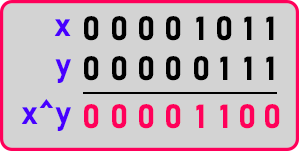In this operation, the result will be 1 if both the bits are different i.e. 0 or 1, 1 or 0. So, the result of x^y is ’0 0 0 0 1 1 0 0’. It is 12 in decimal form.

##### Bitwise NOT Example:

Let us look at NOT:

char x = 5, y;

y = ~x;

We have taken 2 variables ‘x’ with the value of 5 and ‘y’ uninitialized. Then we store ~x in the y variable,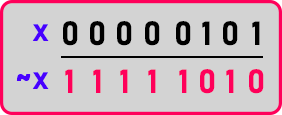Here we know the 1st bit is the sign bit. Now it is 1, which means the number will be negative. So, the result of ‘~x’ is ‘1 1 1 1 1 0 1 0’. For decimal form, we have to convert it into 2’s complement as it is a negative number. So, first, we have to convert 1 to 0 and 0 to 1: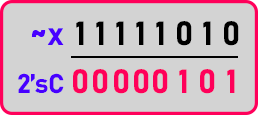We reversed the bit, now we have to add 1: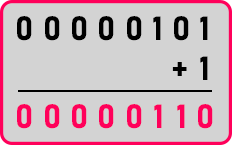Now it is ‘0 0 0 0 0 1 1 0’. It is 6 in decimal form. But as we have sign bit on in ‘~x’ so it will be -6.

##### Where do we need NOT operation?

If you are developing a device driver, or if we are developing code for system programs or system applications or tools then there you may be working closer to electronics and these are the operations in electronics. Your logic itself is defined in terms of bit operations so then you can use this operator for writing your procedures. So, if you are writing hardware-based or device-based drivers then it is useful. Now let us show the left shift and right shift.

##### Left Shift and Right Shift:

int x = 5, y;

y = x << 1;

We want to store the result of the left shift of x by 1 in the y variable. We know the value of x is 5, then in binary will be: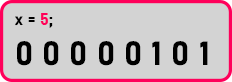In the Left shift, we have to shift all the bits to the left-hand side by the value given by the user. In this case, we will shift all the bits by one to the left-hand side as: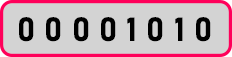When we shift all the bits then the last place will be vacant. So, insert 0 there. Now, this is ‘000001010’ and this is 10 in decimal form. One more important thing is that if we write x << i. (i is some number here then x will be multiplied with 2i. In the right shift, all the bits will be shifted towards the right and the vacant spaces will be filled by ‘0’.We will perform x >> 1 as: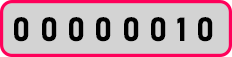If we write x >> i (i is some number here) then x will be divided by 2i. Now it will be 2 in decimal form. Let’s see these all operations in code.

Note: Binary left shift shifts the bits to the left side as the number of times you specified. Mainly it will do multiplication. Binary RightShift shifts the bits to the Right side as the number of times you specified. Mainly it will do division.

##### Bitwise Operators Code in C++ Language:
```#include <iostream>
using namespace std;
int main ()
{
int a = 10, b = 13;
cout << "Bitwise AND: \t" << (a & b) << endl;
cout << "Bitwise OR: \t" << (a | b) << endl;
cout << "Bitwise X-OR: \t" << (a ^ b) << endl;
cout << "Bitwise NOt A: \t" << ~a << endl;
cout << "Bitwise Not B: \t" << ~b << endl;
a = a << 2;
cout << "Bitwise leftshift of a:\t" << a << endl;
b = b >> 2;
cout << "Bitwise Rightshift of b:\t" << b << endl;
return 0;
}
```
###### Output: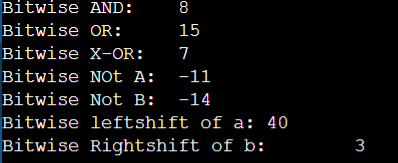In the next article, I am going to discuss Enum and Typedef in C++ with Examples. Here, in this article, I try to explain Bitwise Operators in C++ with Examples and I hope you enjoy this Bitwise Operators in C++ with Examples article.